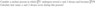# Radioactive decay: U-238 undergoes Alpha and Beta decays and becomes Pb-206

Kim_Jong
Homework Statement:
Consider a nuclear process in which U-238 undergoes several Alpha and Beta decays and becomes Pb-206. How many Alpha and Beta decays occur in this process?
Relevant Equations:
.
Alpha particle has two protons and neutrons so mass = 4.

4x = 238 -206.

x = 1/4(238-206) = 8 Alpha decays.

How would I determine the number of Beta decays? Since the mass doesn't change here.

#### Attachments

•1587392880695.png
7.7 KB · Views: 61
Last edited by a moderator:

•